This blog post outlines a key engineering principle I’ve come to believe strongly in for building general AI systems with deep learning. This principle guides my present-day research tastes and day-to-day design choices in building large-scale, general-purpose ML systems.

Discoveries around Neural Scaling Laws, unsupervised pretraining on Internet-scale datasets, and other work on Foundation Models have pointed to a simple yet exciting narrative for making progress in Machine Learning:

1. Large amounts of diverse data are more important to generalization than clever model biases.
2. If you believe (1), then how much your model generalizes is directly proportional to how fast you can push diverse data into a sufficiently high-capacity model.

To that end, Deep Neural nets trained with supervised learning are excellent data sponges - they can memorize vast amounts of data and can do this quickly by training with batch sizes in the tens of thousands. Modern architectures like ResNets and Transformers seem to have no trouble absorbing increasingly large datasets when trained via supervised learning.

When a model has minimized training loss (a.k.a empirical risk), it can be said to have “memorized” the training set. Classically one would think that minimizing training loss to zero is shortly followed by overfitting, but overparameterized deep networks seem to generalize well even in this regime. Here is an illustration of the “double descent” phenomena from Patterns, Predictions, and Actions, which illustrates that in some problems, overparameterized models can continue to reduce test error (risk) even as training loss is fully minimized.A recent ICLR workshop paper investigates this phenomenon on synthetic datasets, showing that if you train long enough in this zero-training-loss regime, the model can suddenly have an epiphany and generalize much later on (the authors call this “Grokking”). Furthermore, the paper also presents evidence that increasing training data actually decreases the amount of optimization required to generalize.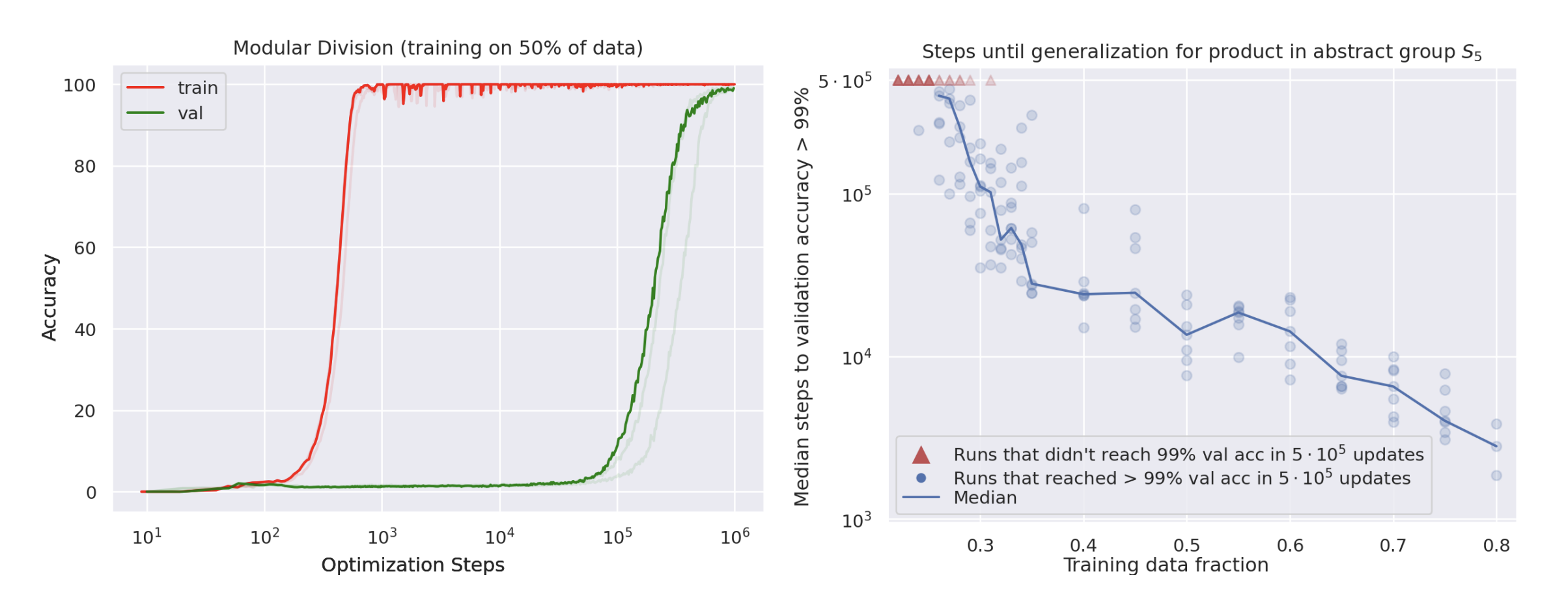It’s as my colleague Chelsea Finn once told me: “Memorization is the first step towards generalization!”

State-of-then-art neural networks trained this way can do really impressive things. Here is a DALL-E model that, when prompted with “A banana performing stand-up comedy”, draws the following picture:Here is another DALL-E output, prompted with “an illstration of a baby panda with headphones staring at its reflection in a mirror”.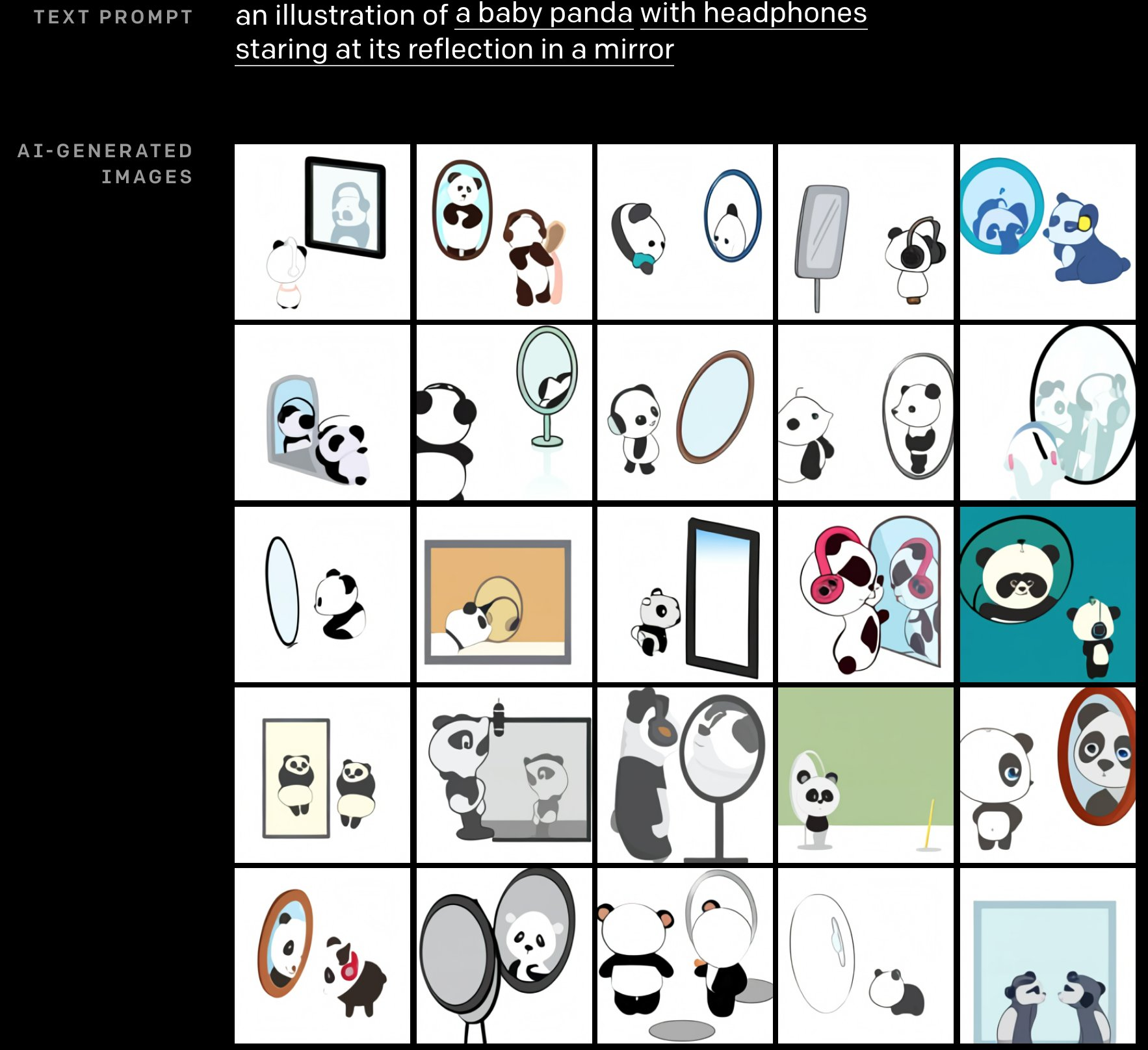Note that there are no such images of “pandas looking into mirrors” or “banana comedians” in the training data (I think), so these results suggest that the DALL-E model has learned to interpret distinct concepts from text, render the corresponding visual parts in an image and have them interact with each other somewhat coherently.

The ability to “just ask” language-conditioned deep learning models for what you want has led to “prompt engineering” as a viable space for improving our ML models. Here is a Tweet discussing how priming a VQGAN + CLIP model with the words “Unreal Engine” leads to drastically higher-quality images.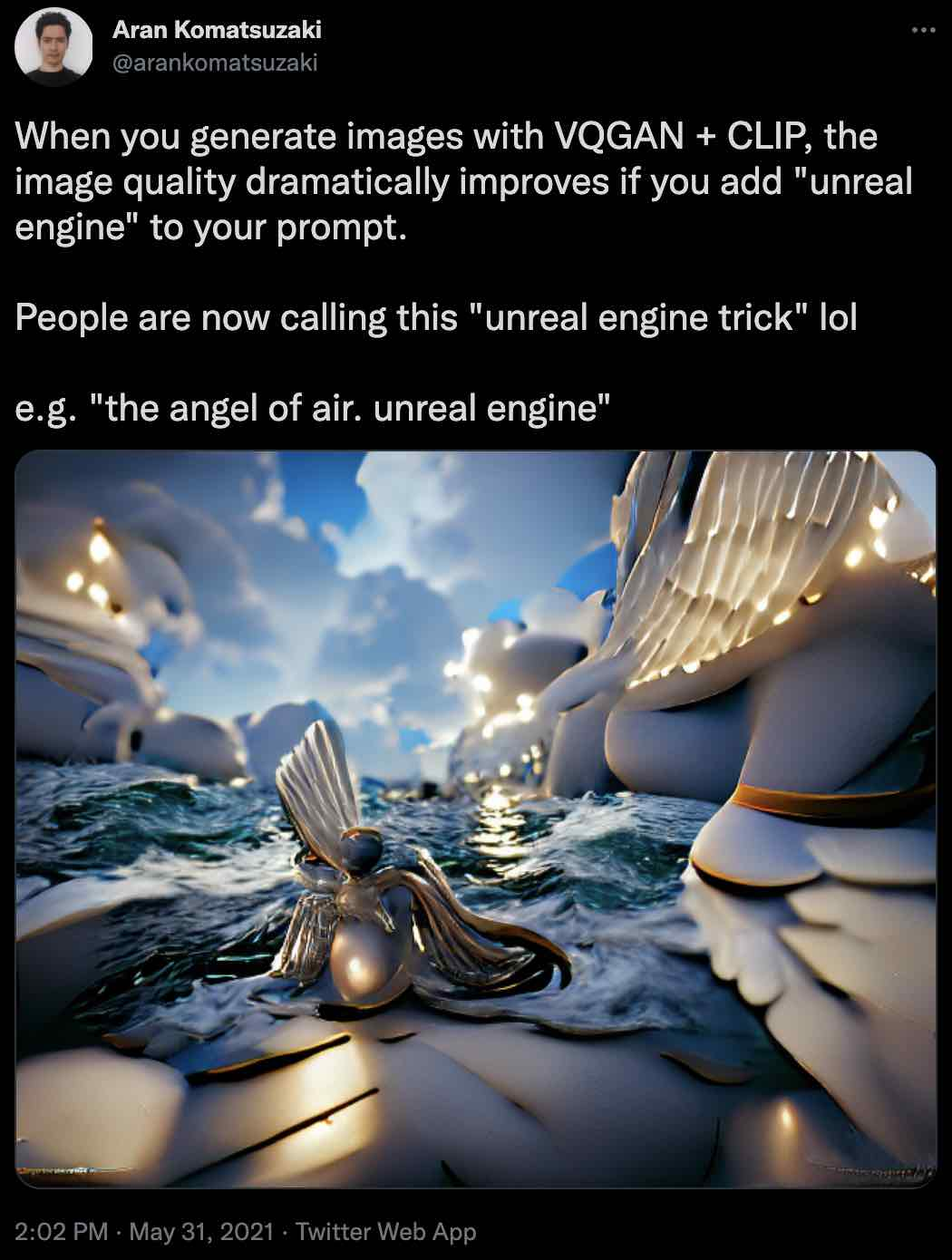What if we could extend this principle - just asking generalization - to other challenging problems that have eluded analytical algorithmic improvements?

# Reinforcement Learning: Not a Great Data Sponge

In contrast to supervised learning, reinforcement learning algorithms are much less computationally efficient when it comes to absorbing vast quantities of diverse data needed for generalization. To see why this is the case, let’s consider a thought experiment where we train a general-purpose robot to do millions of tasks in unstructured environments.

The standard Markov Decision Process is set up as follows: a policy is represented as a state-conditioned distribution over actions, $$p(a \vert s)$$, and the environment as consisting of a reward function $$r(s_t, a_t)$$ and transition dynamics $$p(s_{t+1} \vert s_t, a_t)$$. Initial states and task objectives are encoded in the initial state $$s_0$$, which is sampled from a distribution $$p(s_0)$$. The goal is to maximize the sum of rewards across the episode, averaged across different starting states sampled from $$p(s_0)$$:

$\DeclareMathOperator*{\argmax}{arg\,max} \DeclareMathOperator*{\argmin}{arg\,min} \text{Solve}~\theta^*\ = \argmax_\theta~R(\theta)$ $\text{where}~R(\theta)=E_{p(s_0)}[\sum_{t=1}^{T}{r(s_t, a_t)}]~\text{and}~a_t \sim p_\theta(\cdot|s_t)~\text{and}~s_{t+1} \sim p(\cdot|s_t, a_t)~\text{and}~s_0 \sim p(s_0)$

Let’s assume the existence of some optimal policy which we call $$p^\star(a \vert s)$$, that achieves the maximum reward $$\max_\theta R(\theta)$$. “Supremum” would be more accurate, but I use the $$\max$$ operator for notational simplicity. We want to bring our model, $$p_\theta(a \vert s)$$, as close as possible to $$p^\star(a \vert s)$$.

If we had access to the optimal policy $$p^\star(a \vert s)$$ as an oracle, we could simply query the oracle action and use it like a supervised learning label. We could then train a feedforward policy that maps the states to the oracle actions, and benefit from all the nice properties that supervised learning methods enjoy: stable training, large batches, diverse offline datasets, no need to interact with the environment.

However, in reinforcement learning we often don’t have an expert policy to query, so we must improve the policy from its own collected experience. To do this, estimating the gradient that takes the model policy closer to the optimal policy requires evaluating the average episodic return of the current policy in the environment, and then estimating a gradient of that return with respect to parameters. If you treat the environment returns as a black-box with respect to some parameter $$\theta$$ you can use the log-derivative trick to estimate its gradients:

$\nabla_\theta E_{p(\theta)} [R(\theta)] = \int_\Theta d\theta \nabla_\theta p(\theta) R(\theta) \\ \\ = \int_\Theta d\theta p(\theta) \nabla_\theta \log p(\theta) R(\theta) = E_{p(\theta)} [\nabla_\theta \log p(\theta) R(\theta)]$

This gradient estimator contains two expectations that we need to numerically approximate. First is computing $$R(\theta)$$ itself, which is an expectation over starting states $$p(s_0)$$. In my previous blog post I mentioned that accurate evaluation of a Binomial variable (e.g. the success rate of a robot on a single task) could require thousands of trials in order to achieve statistical certainty within a couple percent. For our hypothetical generalist robot, $$p(s_0)$$ could encompass millions of unique tasks and scenarios, which makes accurate evaluation prohibitively expensive.

The second expectation is encountered in the estimation of the policy gradient, over $$p(\theta)$$. Some algorithms like CMA-ES draw samples directly from the policy parameter distribution $$p(\theta)$$, while other RL algorithms like PPO sample from the policy distribution $$p_\theta(a\vert s)$$ and use the backpropagation rule to compute the gradient of the return with respect to the parameters: $$\frac{\partial R}{\partial \theta} = \frac{\partial R}{\partial \mu_a} \cdot \frac{\partial \mu_a}{\partial \theta}$$. The latter is typically preferred because the search space on action parameters is thought to be smaller than the search space on policy parameters (and therefore requires fewer environment interactions to estimate a gradient for).

If supervised behavior cloning on a single oracle label $$a \sim p^\star(a\vert s)$$ gives you some gradient vector $$g^\star$$, estimating the same gradient vector $$\bar{g} \approx g^\star$$ with reinforcement learning requires something on the order of $$O(H(s_0) \cdot H(a))$$ times as many episode rollouts to get a comparably low-variance estimate. This is a hand-wavy estimate that assumes that there is a multiplicative factor of the entropy of the initial state distribution $$O(H(s_0))$$ for estimating $$R(\theta)$$ and a multiplicative factor of the entropy of the action distribution $$O(H(a))$$ for estimating $$\nabla_\theta R(\theta)$$ itself.

Consequently, online reinforcement learning on sparse rewards and diverse, possibly multi-task environments require enormous numbers of rollouts to estimate returns and their gradients accurately. You have to pay this cost on every minibatch update! When the environment requires handling a wide variety of scenarios and demands generalization to unseen situations, it further increases the number of minibatch elements needed. The OpenAI DOTA team found that having millions of examples in their minibatch was required to bring down gradient noise to an acceptable level. This intuitively makes sense: if your objective $$R(\theta)$$ has a minimum minibatch size needed to generalize well across many $$s_0$$ without excessive catastrophic forgetting, then switching from supervised learning to online reinforcement learning will probably require a larger batch size by some multiplicative factor.

What about offline RL methods like Deep Q-Learning on large datasets of $$(S,A,R,S)$$ transitions? These methods work by bootstrapping, where the target values that we regress value functions to are computed using a copy of the same network’s best action-value estimate on the next state. The appeal of these offline reinforcement learning methods is that you can get optimal policies from diverse, off-policy data without having to interact with the environment. Modified versions of Q-learning like CQL work even better on offline datasets, and have shown promise on smaller-scale simulated control environments.

Unfortunately, bootstrapping does not mix well with generalization. It is folk knowledge that the deadly triad of function approximation, bootstrapping, and off-policy data make training unstable. I think this problem will only get worse as we scale up models and expect to train them on increasingly general tasks. This work shows that repeated bootstrapping iteratively decreases the capacity of the neural network. If you believe the claim that overparameterization of deep neural networks is key to generalization, then it would appear that for the same neural net architecture, offline RL is not quite as “data absorbent” as supervised learning.

In practice, even algorithms like CQL are still challenging to scale and debug on larger, real-world datasets; colleagues of mine tried several variations of AWAC and CQL on large-scale robotics problems and found them to be trickier to get them to work than naive methods like Behavior Cloning.

Instead of going through all this trouble, what if we lean into what deep nets excel at - sponging up data quickly with supervised learning and generalizing to massive datasets? Can we accomplish what RL sets out to do using the tools of generalization, rather than direct optimization?

# Learn the Distribution instead of the Optimum

What if we make generalization the first-class citizen in algorithmic design, and tailor everything else in service of it? What if we could simply learn all the policies with supervised learning, and “just ask nicely” for the best one?

Consider the recent work on Decision Transformer (DT), whereby instead of modeling a single policy and iteratively improving it with reinforcement learning, the authors simply use supervised learning coupled with a sequential model to predict trajectories of many different policies. The model is conditioned with the Return-to-Go so that it may predict actions consistent with a policy that would achieve those returns. The DT simply models all policies - good and bad - with supervised learning, and then use the magic of deep learning generalization to infer from the expert-conditioned policy.

This phenomenon has been observed and developed in several prior and concurrent works, such as Reward-Conditioned Policies, Upside Down Reinforcement Learning and Reinforcement Learning as One Big Sequence Modeling Problem. The AlphaStar team also found that conditioning a model on human player skill level (e.g. future units they ended up build order, MMR, ELO scores) and using it to imitate all player data was superior to only imitating expert-level build orders. This technique is also commonly used in the Autonomous Vehicle space to model both good drivers and bad drivers jointly, even though the autonomous policy is only ever deployed to imitate good driving behavior.

# Hindsight Language Relabeling

At a high level, DTs condition the supervised learning objective on some high level description $$g$$ that partitions what the policy will do in the future based on that value of $$g$$. The return-to-go is an especially salient quantity for a reinforcement learning task, but you can also express the future outcomes via a goal state or StarCraft build order or even a natural language description of what was accomplished.

In Learning Latent Plans from Play, the authors pair arbitrary trajectories with post-hoc natural language descriptions, and then train a model to clone those behaviors conditioned on language. At test time, they simply “ask” the policy to do a novel task in a zero-shot manner. The nice thing about these techniques is that they are indispensable for reaching sparse goals on RL tasks like Ant-Maze. This lends support to the claim that generalization and inference across goal-conditioning can do far better than brute force search for a single sparse goal in a long-horizon task.

Language is a particularly nice choice for conditioning because it can be used to partition a trajectory not just on skill level, but also by task, by how much the policy explores, how “animal-like” it is, and any other observations a human might make about the trajectory. Clauses can be composed ad-hoc without developing a formal grammar for all outcomes that the robot might accomplish. Language is an ideal “fuzzy” representation for the diversity of real-world outcomes and behaviors, which will become increasingly important as we want to partition increasingly diverse datasets.

# Generalizing From Imperfect Demonstrations

A recent work I am quite inspired is D-REX, which tackles the problem of inferring the environment’s reward function from the demonstrations of a suboptimal policy. Classically, one requires making an assumption that the demonstrator is the optimal policy, from which you can use off-policy algorithms (e.g. Q-learning) to estimate the value function. Offline value estimation with deep neural nets can suffer from poor generalization to state-action pairs not in the demonstrator trajectory, and thus requires careful algorithmic tuning to make sure that the value function converges. An algorithm with poor convergence properties makes the propsects of minimizing training loss - and therefore generalization - tenuous. D-REX proposes a really clever trick to get around not having any reward labels at all, even when the demonstrator is suboptimal:

1. Given a suboptimal policy $$\pi_\theta$$, generate trajectory rollouts $$\tau_1, \tau_2, ... \tau_N$$ by having the policy interact with the environment. On each rollout, add variable amounts of noise $$\epsilon$$ to its actions.
2. Assume that adding noise to a suboptimal policy makes it even more suboptimal, i.e. $$R(\tau) \geq R(\tau + \epsilon)$$.
3. Train a ranking model $$f_\theta(\tau_i, \tau_j)$$ to predict which of two trajectories $$\tau_i, \tau_j$$ has a higher return.
4. The ranking model magically extrapolates to trajectories that are better than what $$\pi_\theta$$ can generate, even though the ranking model has never been trained on trajectories better than $$\pi_\theta$$ itself.

I like this approach because ranking models are stable to train (they are just classifiers), and this method is able to achieve better-than-demonstrator behavior not through the explicit construction of the Bellman inequality or implicit planning through a learned model, but rather via extrapolation on a family of perturbations.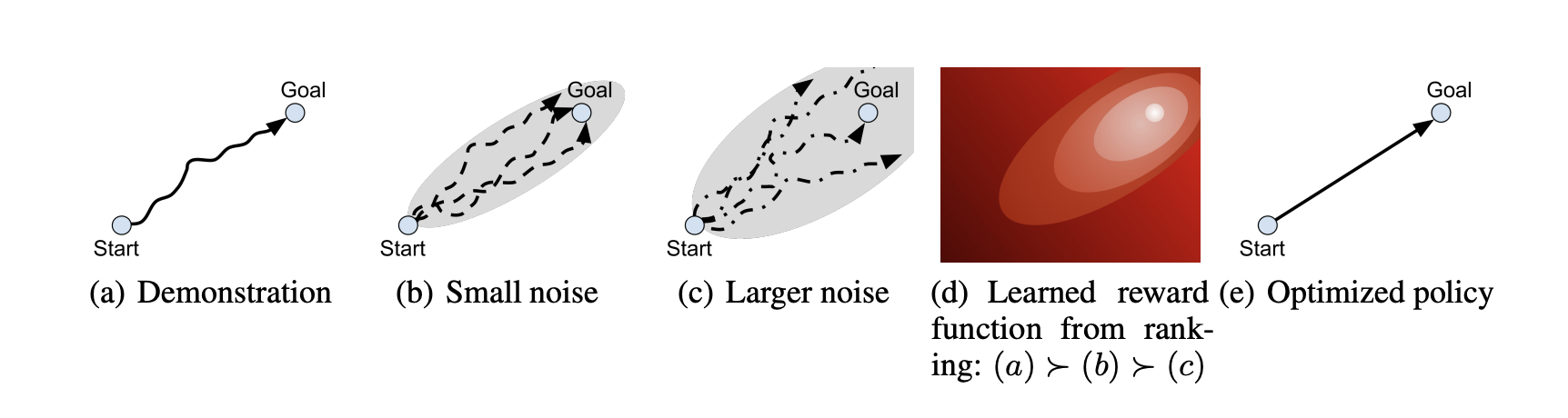# Do You Even Need RL to Improve from Experience?

In the above sections I’ve described how you can “generalize and infer” to get around exploration and even inverse reinforcement learning from sparse rewards. But what about “improving from a policy’s own experience, tabular rasa”? This is the main reason why people put up with the pain of implementing RL algorithms. Can we replace this with supervised learning algorithms and a bit of generalization as well?

The goal of RL is to go from the current set of parameters $$\theta^{n}$$ and some collected policy experience $$\tau$$ to a new set of parameters $$\theta^{n+1}$$ that achieves a higher episode return. Instead of using a “proper” RL algorithm to update the agent, could we just learn this mapping $$f: (\theta^{n}, \tau) \to \theta^{n+1}$$ via supervised deep learning?

This idea is sometimes referred to as “meta-reinforcement learning”, because it involves learning a better reinforcement learning function than off-the-shelf RL algorithms. My colleagues and I applied this idea to a project where we trained a neural network to predict “improved policy behavior” from a video of a lesser policy’s experience. I could imagine this idea being combined with ranking and trajectory augmentation ideas from D-REX to further generalize the “policy improvement behavior”. Even if we never train on optimal policy trajectories, perhaps sufficient data augmentation can also lead to a general improvement operator that extrapolates to the optimal policy regime of parameters.

People often conflate this policy improvement behavior with “reinforcement learning algorithms” like DQN and PPO, but behavior is distinct from implementation. The “policy improvement operator” $$f: (\theta^{n}, \tau) \to \theta^{n+1}$$ can be learned via your choice of reinforcement learning or supervised learning, but is deployed in a RL-like manner for interacting with the environment.

Here is a table summarizing the previously mentioned RL problems, and comparing how each of them can be tackled with a “generalize-and-infer” approach instead of direct optimization.

 Goal “Direct Optimization” Approach “Generalize + Inference” Approach Reinforcement Learning with Sparse Rewards Find $$p^\star(a_t\vert s_t)$$ s.t. $$R_t=1$$, brute force exploration DT: Learn $$p(a_t\vert s_t,R_t)$$ from many policies, infer $$p(a_t\vert s_t, R_t=1)$$. H.E.R - Infer tasks for which gathered trajectories are optimal, then learn $$p(\text{trajectory}\vert \text{task})$$. Then infer optimal trajectory for desired task. Learn a Reward Function from Suboptimal Trajectories Offline Inverse RL D-REX: Trajectory augmentation + Extrapolate to better trajectories. Improve the policy from experience Q-Learning, Policy Gradient Watch-Try-Learn: Learn $$p(\theta^{n+1} \vert \theta^n , \tau, \text{task})$$ Fine-tune a simulated policy in a real-world environment Sample-efficient RL fine-tuning Domain Randomization: train on a distribution of simulators, and the policy “infers which world” it is in at test time.

The high-level recipe is simple. If you want to find the solution $$y_i$$ for a problem $$x_i$$, consider setting up a dataset of paired problems and solutions $$(x_1, y_1), ..., (x_N, y_N)$$ and then training a deep network $$y = f_\theta(x)$$ that “simply maps your problems to solutions”. Then substitute your desired $$x_i$$ and have the deep network infer the solution $$y_i$$ via generalization. “Problem” is meant in the most abstract of terms and can refer to a RL environment, a dataset, or even a single example. “Solutions” could be represented as the optimal parameters of a policy or a neural network, or a single prediction.

Techniques like goal relabeling help generate post-hoc problems from solutions, but building such a dataset can also be achieved via data augmentation techniques. At its core, we are transforming a difficult optimization problem into an inference problem, and training a supervised learning model on a distribution of problems for which it’s comparatively cheap to obtain solutions.

To summarize the recommendations in a three-step recipe:

1. Choose a method capable of minimizing training loss on massive datasets, i.e. supervised learning with maximum likelihood. This will facilitate scaling to complex, diverse datasets and getting the most generalization mileage out of your compute budget.
2. If you want to learn $$p(y\vert x, \text{task}=g^\star)$$ for some prediction task $$g^\star$$, try learning $$p(y\vert x, \text{task})$$ for many related but different tasks $$g \sim p(g), g \neq g^\star$$ Then at test time just condition on $$g^\star$$.
3. Formulate conditioning variables that help partition the data distribution while still admitting generalization on held-out samples from $$p(g)$$. Natural language encoding is a good choice.

The insight that we can cast optimization problems into inference problems is not new. For example, the SGD optimizer can be cast as approximate Bayesian inference and so can optimal control via AICO. These works present a theoretical justification as to why inference can be a suitable replacement for optimization, since the problems and algorithms can be translated back and forth.

I’m suggesting something slightly different here. Instead of casting a sequential decision making problem into an equivalent sequential inference problem, we construct the “meta-problem”: a distribution of similar problems for which it’s easy to obtain the solutions. We then solve the meta-problem with supervised learning by mapping problems directly to solutions. Don’t overthink it, just train the deep net in the simplest way possible and ask it for generalization!

Perhaps in the near future we will be able to prompt-engineer such language-conditioned models with the hint “Generalize to unseen …”.

# Just ask for … Consciousness?

How far can we stretch the principle of “generalize-and-infer” as an alternative to direct optimization? Here is a “recipe for consciousness” which would probably be better pondered over some strong drinks:

1. Train a language-conditioned multi-policy model $$p_\theta(a\vert s, g)$$ (implemented via a Decision Transformer or equivalent) to imitate a variety of policies $$\pi_1, ..., \pi_N$$ conditioned on natural language descriptions $$g$$ of those agents. At test time, some default policy $$p(a\vert s, g=\text{Behave as myself})$$ interacts with another agent $$\pi_\text{test}$$ for a number of steps, after which we instruct the model to “behave as if you were $$\pi_\text{test}$$.” The model would require a sort of “meta-cognition of others” capability, since it would have to infer what policy $$\pi_\text{test}$$ would do in a particular situation.
2. We make a copy of the multi-policy model $$p_\phi \sim p_\theta$$, and embed multiple test-time iterations of step (1) within a single episode, with dozens of agents. Two of these agents are initially conditioned as $$p_\theta(a\vert s, g=\text{Behave as myself})$$ and $$p_\phi(a\vert s, g=\text{Behave as myself})$$. This generates episodes where some agents imitate other agents, and all agents observe this behavior. Then we ask $$p_\phi$$ to emit actions with the conditioning context “behave as if you were $$\pi_\theta$$ pretending to be you”. This would require $$\pi_\phi$$ to model $$\pi_\theta$$’s imitation capabilities, as well as what information $$\pi_\theta$$ knows about $$\pi_\phi$$, on the fly.

Researchers like Jürgen Schmidhuber have previously discussed how dynamics models (aka World Models) of embodied agents are already “conscious”, because successful modeling the dynamics of the environment around oneself necessitates a representation of the self as an embodied participant in the environment.

While I think that “self-representation” is a necessity in planning and dynamics prediction problems, I think the framework is too vacuous to be of use in reproducing a convincing imitation of consciousness. After all, any planning algorithm that represents “the self” explicitly within each imagined trajectory rollout would be conscious under this definition. An A* maze-planner would satisfy this definition of consciousness.

What I’m proposing is implementing a “more convincing” form of consciousness, not based on a “necessary representation of the self for planning”, but rather an understanding of the self that can be transmitted through language and behavior unrelated to any particular objective. For instance, the model needs to not only understand not only how a given policy regards itself, but how a variety of other policies might interpret the behavior of a that policy, much like funhouse mirrors that distort one’s reflection. The hypothesis is that through demonstrating this understanding of “distorted self-reflection”, the policy will learn to recognize itself and model the internal motivations and beliefs of other agents in agent-agent interactions.

There are some important implementation details that I haven’t fleshed out yet, but at high level, I do think that supervised learning and natural language conditioning with enormous agent-interaction datasets are sufficiently powerful tools to learn interesting behaviors. Imbuing agents with some kind of meta-cogition ability of the self and other agents is an important step towards a convincing imitation of consciousness.

# Acknowledgements

Thanks to Daniel Freeman, David Ha, Karol Hausman, Irwan Bello, Igor Mordatch, and Vincent Vanhoucke for feedback and discussion on earlier drafts of this work.

Generalization and scaling:

RL challenges:

Hindsight Imitation

Replacing RL with Supervised Learning

# Q/A

Igor Mordatch supplied interesting questions and comments in reviewing this blog post. I have paraphrased his questions here and added responses in this section.

1. You discussed Supervised Learning and Reinforcement Learning. What do you think about Unsupervised Learning and “The Cake Analogy”?

I consider unsupervised learning to be simply supervised learning for a different task, with comparable gradient variance, since targets are not usually noisly estimated beyond augmentation. Maximum likelihood estimation and contrastive algorithms like InfoNCE seem to be both useful for facilitating generalization in large models.

2. For the first difficulty of RL (evaluating success), aren’t there parallels to current generative models too? Success evaluation is hard for language models, as evidenced by dissatisfaction with BLEU scores and difficulty of evaluating likelihoods with non-likelihood based generative image models.

There are parallels to likelihood-free generative models which require extensive compute for either training or sampling or likelihood evaluation. In practice, however, I think the burdens of evaluation are not directly comparable, since the computational expense of marginalization over observations for such models is dwarfed by the marginalization of success rate estimation in RL. In RL, you have to roll out the environment over O(coin flips) x O(initial state distribution) x O(action distribution) in order to get a low-variance policy gradient for “improved success across all states and tasks”. O(coin flips) is O(1000) samples for local improvement of a couple percent with statistical certainty, wheras I think that typically the marginalization costs of implicit likelihood tends to be cheaper with tricks like Langevin sampling O(minibatch=32). Also, the backprop passes used in Langevin dynamics are usually cheaper than running full environment simulations with a forward pass of the neural net on every step.

3. One of the findings of current language model work is that proxy objectives for what you really want are good enough. Simple next-token prediction induces generalization. But alignment to what you really want is still a hard problem in large model field and we don’t have good answers there yet (and ironically many attempts so far relied on incorporation of RL algorithms).

Alignment objectives may lack a per-example surrogate loss. But under the “generalize-then-infer” school of thought, I would simply recommend learning p(y\vert x, \text{alignment objective}) with max likelihood over numerous hindsight alignment objectives, and then simply condition on the desired alignment object at test time. One could obtain a distribution of alignment descriptions by simply running the model live, and then hindsight labeling with the corresponding alignment realized by the model. Then we simply invoke this meme by Connor Leahy: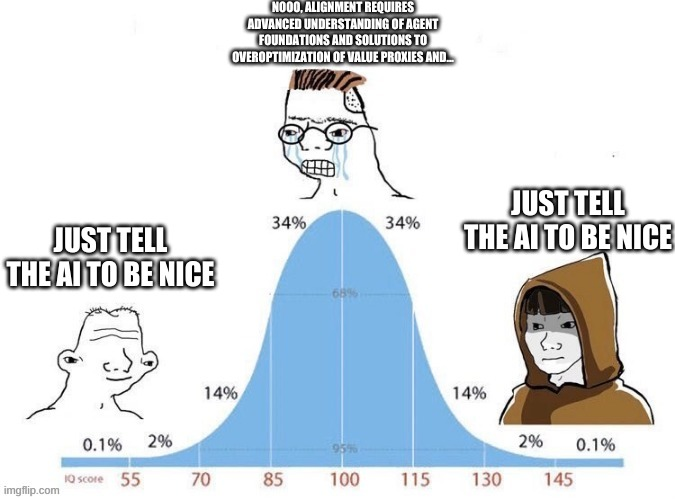Just asking the AI to be nice sounds flippant, but after seeing DALL-E and other large-scale multi-modal models that seem to generalize better as they get bigger, I think we should take these simple, borderline-naive ideas more seriously.

4. For the second difficulty of RL (gradient estimation), we know that for settings where you can backprop through environment dynamics to get exact policy gradient, doing so often leads to worse results.

This reminds me of an old FB comment by Yann Lecun that a better way to estimate Hessian-vector products with ReLU activations is to use a stochastic estimator rather than computing the analytical hessian, since the 2nd-order curvature of ReLU is 0 and what you actually want is the Hessian-vector product of the smoothed version of the function.

If you need to relax the dynamics or use an unbiased stochastic estimator to train through a differentiable simulator, then I think you’re back to where you’re starting with expensive evaluation, since presumably you need many rollouts to smooth out the simulator function and reduce variance. However, maybe the number of samples you need to estimate a smoothed policy gradient is a reasonable tradeoff here and this is a nice way to obtain gradients.

5. Why hasn’t something as simple as what you propose (generalize-then-infer) been done already?

Some researchers out there are probably pursuing this already. My guess is that the research community tends to reward narratives that increase intellectual complexity and argue that “we need better algorithms”. People pay lip service to “simple ideas” but few are willing to truly pursue simplicity to its limit and simply scale up existing ideas.

Another reason would be that researchers often don’t take generalization for granted, so it’s often quicker to think about adding explicit inductive biases rather than thinking about generalization as a first-class citizen and then tailoring all other design decisions in support of it.

6. How does your consciousness proposal relate to ideas from Schmidhuber’s “consciousness in world models” ideas, Friston’s Free Energy Principle, and Hawkin’s “memory of thoughts”?

I consider Schmidhuber and Friston’s unified theories as more or less stating “optimal control requires good future prediction and future prediction with me in it requires self-representation”. If we draw an analogy to next-word prediction in large language models, maybe optimizing next state prediction perfectly is sufficient for subsuming all consciousness-type behaviors like theory-of-mind and the funhouse self-reflections I mentioned above. However, this would require an environment where predicting such dynamics accurately has an outsized impact on observation likelihoods. One critique I have about Schmidhuber and Friston’s frameworks is that they are too general, and can be universally applied to sea slugs and humans. If a certain environmental complexity is needed for future prediction to give rise to something humans would accept as conscious, then the main challenge is declaring what the minimum complexity would be.

Hawkin’s “consciousness as memory of perception” seems to be more related to the subjective qualia aspect of consciousness rather than theory of mind. Note that most people do not consider a program that concatenates numpy arrays to be capable of “experiencing qualia” in the way humans do. Perhaps what is missing is the meta-cognition aspect - the policy needs to exhibit behaviors suggesting that it contemplates the fact that it experiences things. Again, this requires a carefully designed environment that demands such meta-cognition behavior.

I think this could emerge from training for the theory-of-mind imitation problems I described above, since the agent would need to access a consistent representation about how it perceives things and transform it through a variety of “other agent’s lenses”. The flexibility of being able to project one’s own representation of sensory observations through one’s representation of other agents’ sensory capabilities is what would convince me that the agent understands that it can do sufficient meta-cognition about qualia.

7. Your formulation of consciousness only concerns itself with theory-of-mind behavior. What about attention behavior?

See the second paragraph of the response to #6.

Update 20211025: Updated with a paraphrased question from Alexander Terenin

8. In Rich Sutton’s Bitter Lesson Essay, he argues that search and learning are both important. Do you really think that search can be completely replaced by a learned approach?

I agree that having a bit of light search in your program can be immensely helpful to learning and overall performance. It’s a bit of a chicken/egg though. Does AlphaGo work because MCTS uses a learned value function to make search tractable? Or does the policy distillation only work because of search? I’m suggesting that when search becomes too hard (most RL tasks), it’s time to use more learning. You’re still doing search when performing supervised learning - you just get a lot more gradient signal per flop of computation.